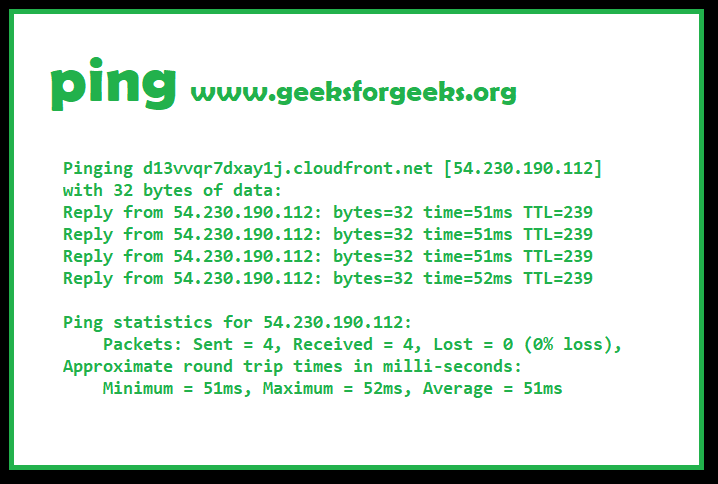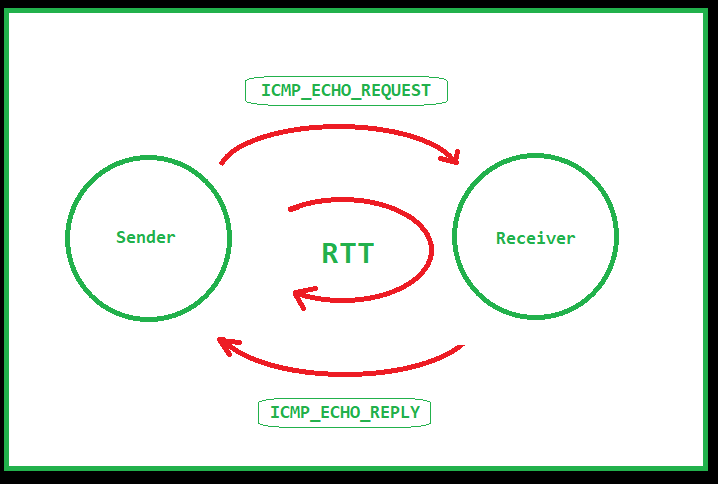Skip to content
Related Articles
Ping in C
• Difficulty Level : Hard
• Last Updated : 07 Jun, 2018

Prerequisites : ICMP | Raw Socket | Internet Checksum | DNS

Ping is a necessity for debugging of the Internet.
Ping is a basic Internet tool that allows a user to verify that a particular IP address exists and can accept requests., with other facilities.Ping sends out ICMP packets by opening a RAW socket, which is separate from TCP and UDP.

Since IP does not have any inbuilt mechanism for sending error and control messages. It depends on Internet Control Message Protocol (ICMP) to provide an error control. It is used for reporting errors and management queries.

Example of Ubuntu Ping

```ping www.google.com
PING www.google.com (172.217.194.105) 56(84) bytes of data.
64 bytes from 172.217.194.105 (172.217.194.105): icmp_seq=1 ttl=46 time=116 ms
64 bytes from 172.217.194.105 (172.217.194.105): icmp_seq=2 ttl=46 time=102 ms
64 bytes from 172.217.194.105 (172.217.194.105): icmp_seq=3 ttl=46 time=119 ms
^C
--- www.google.com ping statistics ---
3 packets transmitted, 3 received, 0% packet loss, time 3110ms

```

Working Mechanism
The Internet Ping program works much like a sonar echo-location, sending a small packet of information containing an ICMP ECHO_REQUEST to a specified computer, which then sends an ECHO_REPLY packet in return.
The packet has a TTL (time-to-live) value determining max number of router hops.

If the packet does not reach, then the sender is noted back with the error.

Errors are of following types:

• TTL Expired in Transit
• Destination Host Unreachable
• Request Timed Out i.e. no reply
• Unknown HostImplementation
The steps followed by a simple ping program are:

1. Take a hostname as input
2. Do a DNS lookup

DNS lookup can be done using gethostbyname(). The gethostbyname() function converts a normal human readable website and returns a structure of type hostent which contains IP address in form of binary dot notation and also address type.

3. Some ping programs like the one given with ubuntu support reverse DNS lookup.
Reverse DNS lookup is performed using getnameinfo(), and it converts dot notation IP address to hostname.

for example, the pinging of google.com frequently gives a strange address:
bom07s18-in-f14.1e100.net

This is as a result of a reverse DNS lookup.

4. Open a Raw socket using SOCK_RAW with protocol as IPPROTO_ICMP.
Note: raw socket requires superuser rights so you have to run this code using sudo
5. When crtl + C is pressed, ping gives a report. This interrupt is caught by an interrupt handler
which just sets our pinging looping condition to false.
6. Here comes the main ping sending loop.
We have to:
1. Set the ttl option to a value in the socket
TTL value is set to limit the number of hops a packet can make.
2. Set the timeout of the recv function
If timeout is not set, recv will wait forever, halting the loop.
3. Fill up the icmp packet
As follows:
1. Set packet header type to ICMP_ECHO.
2. Set id to pid of process
3. Fill msg part randomly.
4. Calculate checksum and fill it in checksum field.
4. Send the packet
5. Wait for it to be received.
The main problem here is that the packet received does not mean that that the destination is working.
Echo reply means destination is OK. Echo reply is sent from destination OS kernel.

This is the list of all types and codes.
A issue here is that the program shows type 69 and code 0 if all goes correct instead of 0 which stands for echo_reply.

 `// C program to Implement Ping`` ` `// compile as -o ping``// run as sudo ./ping `` ` `#include ``#include ``#include ``#include ``#include ``#include ``#include ``#include ``#include ``#include ``#include ``#include ``#include ``#include `` ` `// Define the Packet Constants``// ping packet size``#define PING_PKT_S 64``  ` `// Automatic port number``#define PORT_NO 0 `` ` `// Automatic port number``#define PING_SLEEP_RATE 1000000 x`` ` `// Gives the timeout delay for receiving packets``// in seconds``#define RECV_TIMEOUT 1 `` ` `// Define the Ping Loop``int` `pingloop=1;`` ` ` ` `// ping packet structure``struct` `ping_pkt``{``    ``struct` `icmphdr hdr;``    ``char` `msg[PING_PKT_S-``sizeof``(``struct` `icmphdr)];``};`` ` `// Calculating the Check Sum``unsigned ``short` `checksum(``void` `*b, ``int` `len)``{    unsigned ``short` `*buf = b;``    ``unsigned ``int` `sum=0;``    ``unsigned ``short` `result;`` ` `    ``for` `( sum = 0; len > 1; len -= 2 )``        ``sum += *buf++;``    ``if` `( len == 1 )``        ``sum += *(unsigned ``char``*)buf;``    ``sum = (sum >> 16) + (sum & 0xFFFF);``    ``sum += (sum >> 16);``    ``result = ~sum;``    ``return` `result;``}`` ` ` ` `// Interrupt handler``void` `intHandler(``int` `dummy)``{``    ``pingloop=0;``}`` ` `// Performs a DNS lookup ``char` `*dns_lookup(``char` `*addr_host, ``struct` `sockaddr_in *addr_con)``{``    ``printf``(``"\nResolving DNS..\n"``);``    ``struct` `hostent *host_entity;``    ``char` `*ip=(``char``*)``malloc``(NI_MAXHOST*``sizeof``(``char``));``    ``int` `i;`` ` `    ``if` `((host_entity = gethostbyname(addr_host)) == NULL)``    ``{``        ``// No ip found for hostname``        ``return` `NULL;``    ``}``     ` `    ``//filling up address structure``    ``strcpy``(ip, inet_ntoa(*(``struct` `in_addr *)``                          ``host_entity->h_addr));`` ` `    ``(*addr_con).sin_family = host_entity->h_addrtype;``    ``(*addr_con).sin_port = htons (PORT_NO);``    ``(*addr_con).sin_addr.s_addr  = *(``long``*)host_entity->h_addr;`` ` `    ``return` `ip;``     ` `}`` ` `// Resolves the reverse lookup of the hostname``char``* reverse_dns_lookup(``char` `*ip_addr)``{``    ``struct` `sockaddr_in temp_addr;    ``    ``socklen_t len;``    ``char` `buf[NI_MAXHOST], *ret_buf;`` ` `    ``temp_addr.sin_family = AF_INET;``    ``temp_addr.sin_addr.s_addr = inet_addr(ip_addr);``    ``len = ``sizeof``(``struct` `sockaddr_in);`` ` `    ``if` `(getnameinfo((``struct` `sockaddr *) &temp_addr, len, buf, ``                    ``sizeof``(buf), NULL, 0, NI_NAMEREQD)) ``    ``{``        ``printf``(``"Could not resolve reverse lookup of hostname\n"``);``        ``return` `NULL;``    ``}``    ``ret_buf = (``char``*)``malloc``((``strlen``(buf) +1)*``sizeof``(``char``) );``    ``strcpy``(ret_buf, buf);``    ``return` `ret_buf;``}`` ` `// make a ping request``void` `send_ping(``int` `ping_sockfd, ``struct` `sockaddr_in *ping_addr,``                ``char` `*ping_dom, ``char` `*ping_ip, ``char` `*rev_host)``{``    ``int` `ttl_val=64, msg_count=0, i, addr_len, flag=1,``               ``msg_received_count=0;``     ` `    ``struct` `ping_pkt pckt;``    ``struct` `sockaddr_in r_addr;``    ``struct` `timespec time_start, time_end, tfs, tfe;``    ``long` `double` `rtt_msec=0, total_msec=0;``    ``struct` `timeval tv_out;``    ``tv_out.tv_sec = RECV_TIMEOUT;``    ``tv_out.tv_usec = 0;`` ` `    ``clock_gettime(CLOCK_MONOTONIC, &tfs);`` ` `     ` `    ``// set socket options at ip to TTL and value to 64,``    ``// change to what you want by setting ttl_val``    ``if` `(setsockopt(ping_sockfd, SOL_IP, IP_TTL, ``               ``&ttl_val, ``sizeof``(ttl_val)) != 0)``    ``{``        ``printf``("\nSetting socket options ``                 ``to TTL failed!\n");``        ``return``;``    ``}`` ` `    ``else``    ``{``        ``printf``(``"\nSocket set to TTL..\n"``);``    ``}`` ` `    ``// setting timeout of recv setting``    ``setsockopt(ping_sockfd, SOL_SOCKET, SO_RCVTIMEO,``                   ``(``const` `char``*)&tv_out, ``sizeof` `tv_out);`` ` `    ``// send icmp packet in an infinite loop``    ``while``(pingloop)``    ``{``        ``// flag is whether packet was sent or not``        ``flag=1;``      ` `        ``//filling packet``        ``bzero(&pckt, ``sizeof``(pckt));``         ` `        ``pckt.hdr.type = ICMP_ECHO;``        ``pckt.hdr.un.echo.id = getpid();``         ` `        ``for` `( i = 0; i < ``sizeof``(pckt.msg)-1; i++ )``            ``pckt.msg[i] = i+``'0'``;``         ` `        ``pckt.msg[i] = 0;``        ``pckt.hdr.un.echo.sequence = msg_count++;``        ``pckt.hdr.checksum = checksum(&pckt, ``sizeof``(pckt));`` ` ` ` `        ``usleep(PING_SLEEP_RATE);`` ` `        ``//send packet``        ``clock_gettime(CLOCK_MONOTONIC, &time_start);``        ``if` `( sendto(ping_sockfd, &pckt, ``sizeof``(pckt), 0, ``           ``(``struct` `sockaddr*) ping_addr, ``            ``sizeof``(*ping_addr)) <= 0)``        ``{``            ``printf``(``"\nPacket Sending Failed!\n"``);``            ``flag=0;``        ``}`` ` `        ``//receive packet``        ``addr_len=``sizeof``(r_addr);`` ` `        ``if` `( recvfrom(ping_sockfd, &pckt, ``sizeof``(pckt), 0, ``             ``(``struct` `sockaddr*)&r_addr, &addr_len) <= 0``              ``&& msg_count>1) ``        ``{``            ``printf``(``"\nPacket receive failed!\n"``);``        ``}`` ` `        ``else``        ``{``            ``clock_gettime(CLOCK_MONOTONIC, &time_end);``             ` `            ``double` `timeElapsed = ((``double``)(time_end.tv_nsec - ``                                 ``time_start.tv_nsec))/1000000.0``            ``rtt_msec = (time_end.tv_sec-``                          ``time_start.tv_sec) * 1000.0``                        ``+ timeElapsed;``             ` `            ``// if packet was not sent, don't receive``            ``if``(flag)``            ``{``                ``if``(!(pckt.hdr.type ==69 && pckt.hdr.code==0)) ``                ``{``                    ``printf``("Error..Packet received with ICMP ``                           ``type %d code %d\n", ``                           ``pckt.hdr.type, pckt.hdr.code);``                ``}``                ``else``                ``{``                    ``printf``("%d bytes from %s (h: %s) ``                          ``(%s) msg_seq=%d ttl=%d ``                          ``rtt = %Lf ms.\n", ``                          ``PING_PKT_S, ping_dom, rev_host, ``                          ``ping_ip, msg_count,``                          ``ttl_val, rtt_msec);`` ` `                    ``msg_received_count++;``                ``}``            ``}``        ``}    ``    ``}``    ``clock_gettime(CLOCK_MONOTONIC, &tfe);``    ``double` `timeElapsed = ((``double``)(tfe.tv_nsec - ``                          ``tfs.tv_nsec))/1000000.0;``     ` `    ``total_msec = (tfe.tv_sec-tfs.tv_sec)*1000.0+ ``                          ``timeElapsed``                    ` `    ``printf``(``"\n===%s ping statistics===\n"``, ping_ip);``    ``printf``("\n%d packets sent, %d packets received, %f percent``           ``packet loss. Total ``time``: %Lf ms.\n\n", ``           ``msg_count, msg_received_count,``           ``((msg_count - msg_received_count)/msg_count) * 100.0,``          ``total_msec); ``}`` ` `// Driver Code``int` `main(``int` `argc, ``char` `*argv[])``{``    ``int` `sockfd;``    ``char` `*ip_addr, *reverse_hostname;``    ``struct` `sockaddr_in addr_con;``    ``int` `addrlen = ``sizeof``(addr_con);``    ``char` `net_buf[NI_MAXHOST];`` ` `    ``if``(argc!=2)``    ``{``        ``printf``(``"\nFormat %s
\n"``, argv);``        ``return` `0;``    ``}`` ` `    ``ip_addr = dns_lookup(argv, &addr_con);``    ``if``(ip_addr==NULL)``    ``{``        ``printf``("\nDNS lookup failed! Could ``                   ``not resolve hostname!\n");``        ``return` `0;``    ``}`` ` `    ``reverse_hostname = reverse_dns_lookup(ip_addr);``    ``printf``(``"\nTrying to connect to '%s' IP: %s\n"``,``                                       ``argv, ip_addr);``    ``printf``(``"\nReverse Lookup domain: %s"``, ``                           ``reverse_hostname);`` ` `    ``//socket()``    ``sockfd = socket(AF_INET, SOCK_RAW, IPPROTO_ICMP);``    ``if``(sockfd<0)``    ``{``        ``printf``(``"\nSocket file descriptor not received!!\n"``);``        ``return` `0;``    ``}``    ``else``        ``printf``(``"\nSocket file descriptor %d received\n"``, sockfd);`` ` `    ``signal``(SIGINT, intHandler);``//catching interrupt`` ` `    ``//send pings continuously``    ``send_ping(sockfd, &addr_con, reverse_hostname, ``                                 ``ip_addr, argv);``     ` `    ``return` `0;``}`

An example output:
Run sudo ./ping google.com

```Resolving DNS..

Trying to connect to 'google.com' IP: 172.217.27.206

Reverse Lookup domain: bom07s15-in-f14.1e100.net
Socket file descriptor 3 received

Socket set to TTL..
64 bytes from bom07s15-in-f14.1e100.net (h: google.com) (172.217.27.206)
msg_seq=1 ttl=64 rtt = 57.320584 ms.

64 bytes from bom07s15-in-f14.1e100.net (h: google.com) (172.217.27.206)
msg_seq=2 ttl=64 rtt = 58.666775 ms.

64 bytes from bom07s15-in-f14.1e100.net (h: google.com) (172.217.27.206)
msg_seq=3 ttl=64 rtt = 58.081148 ms.

64 bytes from bom07s15-in-f14.1e100.net (h: google.com) (172.217.27.206)
msg_seq=4 ttl=64 rtt = 58.700630 ms.

64 bytes from bom07s15-in-f14.1e100.net (h: google.com) (172.217.27.206)
msg_seq=5 ttl=64 rtt = 58.281802 ms.

64 bytes from bom07s15-in-f14.1e100.net (h: google.com) (172.217.27.206)
msg_seq=6 ttl=64 rtt = 58.360916 ms.

===172.217.27.206 ping statistics===

6 packets sent, 6 packets received, 0.000000 percent packet loss.
Total time: 6295.187804 ms.
```

Attention reader! Don’t stop learning now. Get hold of all the important CS Theory concepts for SDE interviews with the CS Theory Course at a student-friendly price and become industry ready.

My Personal Notes arrow_drop_up